# Why does Prof. Loh multiply r minus d with d plus r?

• Module 2 Week 2 Day 7 Challenge Explanation Part 4

So it is said that in the Power of a Point theorem, PA times PC equals PB times PD. However, why does Prof. Loh multiply r minus d with d plus r at around timestamp 13:25 instead of just d times r? Also could you please clarify what the Power of a Point theorem is? I seem to see different variations.

• And where does 9 times 21 come from in the end? Also what does that whole other thing about r and d have to do with 9 times 21? Aren't they different?

• Hi TSS Graviser,

That was a slick thing that Prof. Loh did! Sort of like a sleight-of-hand. The point that is the center of the circle looks like it might have been the endpoint of the line segments he was using for Power of a Point, but it wasn't. This is why it's a bit confusing.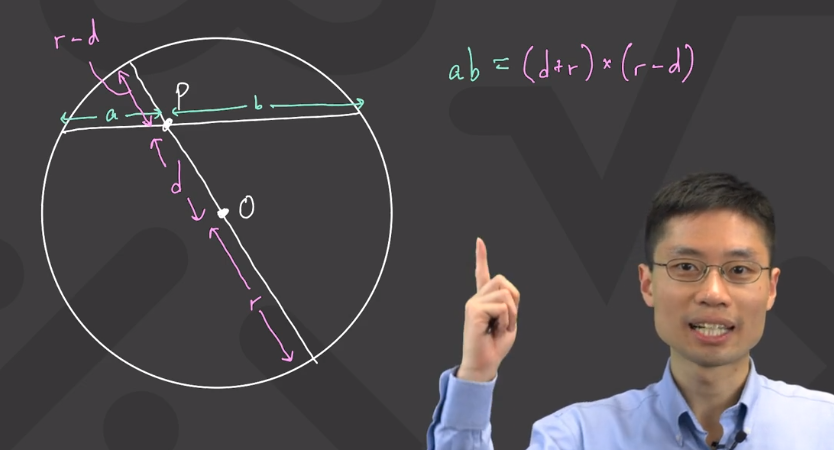We created the variable $$d$$ because we happen to want to solve for that distance from the actual intersection point to the center of the circle. It's a bit confusing that $$d$$ isn't a length of a segment that we'll use for Power of Point. However, the reason we do this is because we will get a neat difference of squares, which will allow us to isolate out the variable $$d.$$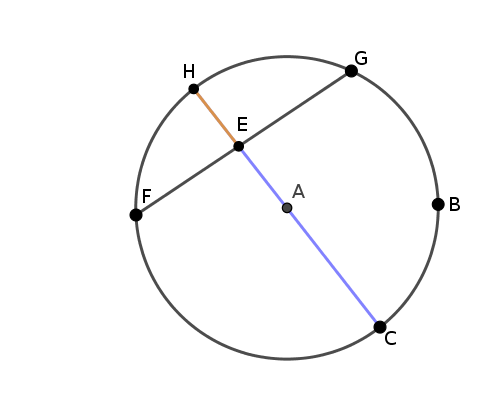See that in the above diagram, we can use Power of a Point to say that

$$\text{orange length} \times \text{purple length} = \overline{FE} \times \overline{EG}$$

If we call the length of $$\overline{EA} \text{ } d$$, then the orange length is equal to $$r - d,$$ and the purple length is equal to $$r + d.$$

When we multiply out the orange length and the purple length, we get

$$(r-d) \times (r+d)$$

which equals $$r^2 - d^2.$$

This is very nice! If we know the radius of the circle, then we can solve for the distance $$d.$$

Happy Learning!

• What is the power of a point theorem?

• @TSS-Graviser The Power of a Point Theorem seems to take several forms, depending on whether the point in question is inside the circle or outside, and depending on how the lines connecting the point intersect the circle: tangent (at one point only) or secant (in two points).

In the screenshot above, we are using an instance of an interior point inside a circle.

You can also extend Power of a Point to points outside the circle.
For example, you can use the Power of a Point Theorem on the point $$A$$ below to see that $$\overline{AF} \times \overline{AE} = \overline{AB} \times \overline{AD}.$$ In this case, $$A$$ is an exterior point, and it is connected by secant lines to the circle. You can also use Power of a Point on point $$C$$ to see that $$\overline{CB}^2 = \overline{CE} \times \overline{CF}.$$ In this case, $$C$$ is connected by one tangent and one secant line to the circle.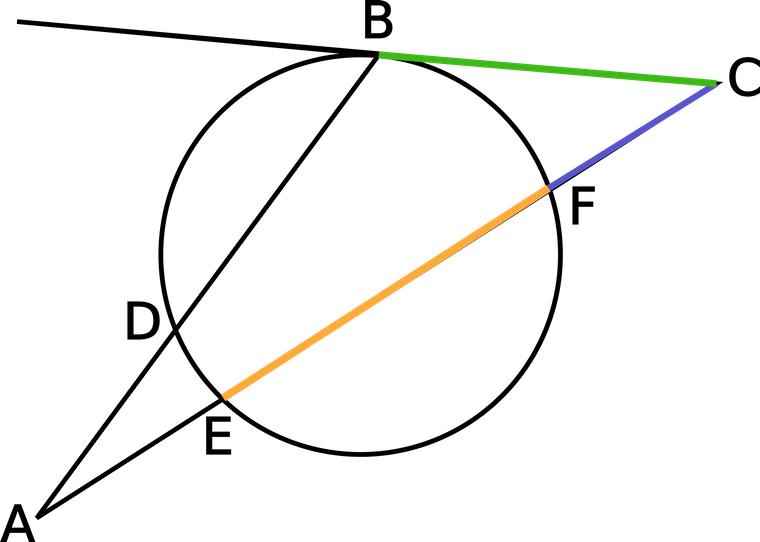Why do we call these all "Power of a Point"? It's because the method of proving the relationships is very much the same: find angles that are the same measure based on which arcs of the circle they inscribe, and use these same angles to find similar triangles. Once you have the similar triangles, express proportions between the matching sides. Then fiddle around to get rid of the fraction and make it into the form that we're all familiar with!

• How do we know that some of these lines' lengths multiplied together have the same product as the products of another set of lines? Is it because they both share the same endpoint and do not cross outside of the circle? But won't distances still differ then?

• @TSS-Graviser It's really great that you want to understand why this formula works. (And practically speaking, being able to prove why theorems are true helps you remember them!) Starting from around timestamp 6:00 in the Day 7 Challenge Explanation, Prof. Loh starts proving Power of a Point, for the case of a point being inside the circle. Remember how I mentioned the proof involves similar triangles?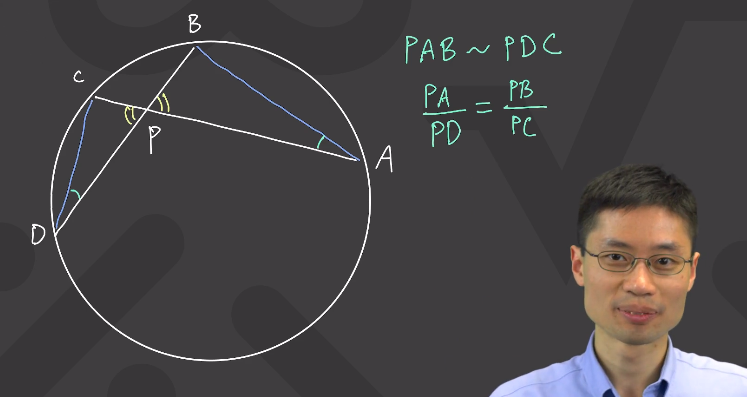In this screenshot, you see that $$\angle CPD$$ is the same as angle $$\angle BPA$$ because they are formed from the "X." Also, we know that the green marked angles, $$\angle CDP$$ and $$\angle BAP,$$ are the same. Why are they the same? Because they both open up to, or inscribe, the same arc, the one that is between points C and B. Wow! So we have two angles the same in both triangles; that must mean that all of their angles are the same. So the triangles are similar!

Now this is nice, because the sides of the triangles are the line segments that we want to use for Power of a Point. We can sort of smell the theorem already. But first, we need to sort out which sides are the same on both triangles.

Try imagining that you could fold the screen at the point P, making the lines of the triangles overlap each other. $$\overline{PA}$$ would rest on top of $$\overline{PD},$$ and $$\overline{PB}$$ would rest on top of $$\overline{PC}.$$

Now do you see how Prof. Loh got the equation

$$\frac{\overline{PA}}{\overline{PD}} = \frac{\overline{PB}}{\overline{PC}}$$

?

And do you see that if you multiply both sides by $$\overline{PD}$$ and $$\overline{PC},$$ you get the Power of a Point Theorem?

$$\overline{PA} \times \overline{PC} = \overline{PB} \times \overline{PD}$$

In order to find the equivalent relation for the case when a point is outside the circle, you do a similar proof. You find similar triangles based on the angles that inscribe the same arcs, etc.

The great thing about understanding the proof is that if you ever forget the theorem, you can just derive it again whenever you need it!• Do angles have to do with the length of a line segment in contrast to another line segment altogether in contrast to another two sets of line segments? Like for example PA to PB vs PC to PD. Do angles determine the ratios?

• @TSS-Graviser It's kind of neat that all of your questions about angles, arcs and Power of a Point Theorem are tying in together! Now I see that you're trying to synthesize it in your head in order to make it into a tool for yourself to use. It's very good to ask, "When exactly can I use this? When can I not use this?" If you don't mind, I'll take the long approach again and backtrack to the proof of Power of a Point and how it all ties in together.

First, let's start with the two chords that intersect in a circle.
The yellow angle inscribes the yellow arc here:Here, with the second chord, we can connect its angle vertex in such a way that the same arc is inscribed by the second yellow angle.Now it's time to look at the other angles of the triangles! There are two other angles that inscribe the same arc of the circle, the green ones shown here: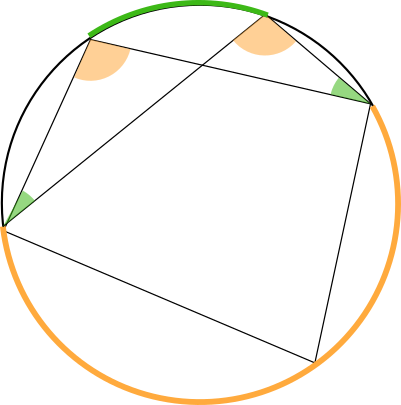And we also know that the angles formed by the "X" are the same, so the two triangles drawn inside the circle are similar! (They're not necessarily congruent, because we don't know any of the lengths.)

These similar triangles are analogous to the similar triangles that we saw in the Day 7 Challenge video: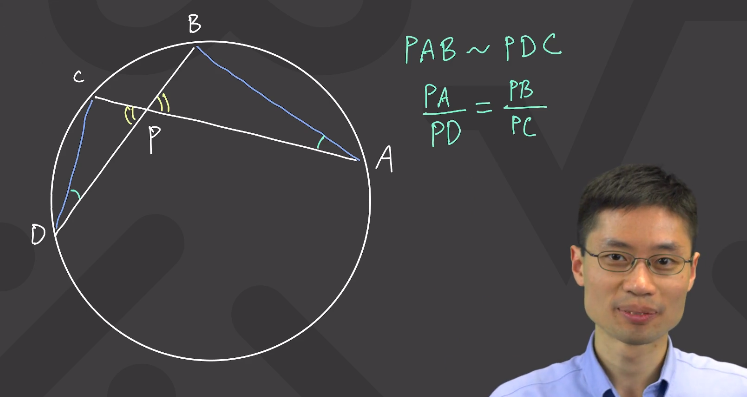The sides $$\overline{PA}$$ and $$\overline{PD}$$ are matching sides that go from $$\angle \text{yellow}$$ to $$\angle \text{green}$$, and the sides $$\overline{PB}$$ and $$\overline{PC}$$ are matching sides that go from $$\angle \text{yellow}$$ to the third angle (not colored). This is the reason for writing the ratios

$$\frac{ PA}{PD} = \frac{PB}{PC}$$

If this looks confusing, perhaps try to imagine that the large triangle is twice the dimensions of the small triangle. Then $$\overline{PA}$$ would be double $$\overline{PD},$$ and $$\overline{PB}$$ would be double $$\overline{PC}.$$ Then

$$\frac{ PA}{PD} = \frac{PB}{PC} = 2$$

At any rate, if you multiply both sides by $$\overline{PD}$$ and $$\overline{PC},$$ you can cancel the denominators to get the Power of a Point theorem:

$$PA \times PC = PD \times PB$$

What's the pattern that we can find in this formula? Well, $$\overline{PA}$$ and $$\overline{PC}$$ are on the same chord, $$\overline{CA},$$ and $$\overline{PD}$$ and $$\overline{PB}$$ are on the same chord $$\overline{BD}.$$ In other words, try to look for the entire chords in the picture; their segments will be the ones that you multiply together in Power of a Point. The purple line below is one chord: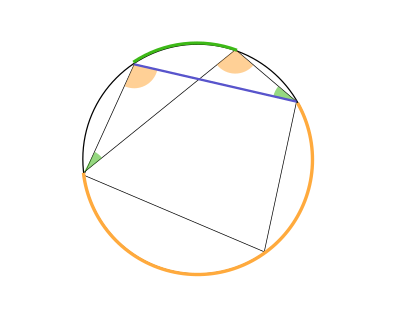And this purple line is another: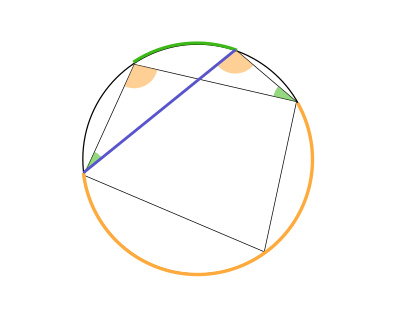Now, here is the tool we have for applying the Power of a Point Theorem:

• Take the two segments of one chord, and multiply them together.

• Take the two segments of the other chord, and multiply them together.

• These two products should equal each other.

I hope this made sense! Let me know!• Sorry I get the first part now but I don't get all those angle equations in the second half. I just want to know if angles determine side length relationships.

• @TSS-Graviser Oh, are you referring to the equations like

$$\frac{PA}{PD} = \frac{PB}{PC}?$$

I think I might see what you're confused about. Are you thinking that $$PA, PD, PB,$$ and $$PC$$ are measures of angles? They are actually lengths of lines segments.

If we were talking about the measure of angles, we would write this as

$$\angle CPD$$

for the yellow angle, for example.

By the way, if you want to write this yourself, you can type

\$$\angle CPD \$$• No I mean all that matching stuff and whatnot. Is there like a simplified explanation that like I can digest?

• And by the way, when can power of a point be used? It seems that I can use it anywhere with intersections, but is that always the case?

• @TSS-Graviser Oh, I see! Do you mean that you're not sure how to figure out which sides match? Try imagining that you are folding the screen like closing a book, so that the yellow angles lie on top of each other and the green angles also lie on top of each other. Do you see that $$\overline{PA}$$ will lie right on top of $$\overline{PD}?$$

• @debbie Yes! Whenever you have two chords intersecting inside a circle, you can use Power of a Point.• @TSS-Graviser The simplified version was found at the bottom of the earlier very long reply:

Now, here is the tool we have for applying the Power of a Point Theorem:

Take the lengths of two segments of one chord, and multiply them together.

Take the lengths of two segments of the other chord, and multiply them together.

These two products should equal each other.

I hope this made sense! Let me know!• Oh ok thanks. What I've been trying to say is that above in the pictures with the arcs, right, how do all these line segments on the same chord mean anything? And what's the point of labeling the orange arc and the green arc? Do they have a purpose?

• @TSS-Graviser That's a good question; it depends on what you mean by meaningful! The line segments happen to be the side lengths of the triangles in the picture. Maybe you will see a math question that asks for one of the lengths of the triangles. In that case, knowing Power of a Point will be very meaningful!

The yellow arc goes with the yellow angle. The yellow angle is inscribed inside the yellow arc. That means that the yellow angle's measure is $$\frac{1}{2}$$ of the measure of the arc.

So to answer your emailed question,, suppose an angle opens up to exactly half of the circle. That half of the circle is an arc with measure $$180^{\circ}.$$ That means the angle is $$90^{\circ}.$$